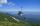Height difference

What height difference overcome if we pass road 1 km long with a pitch21 per mille?

Result

x =  20.995 m

Solution:Leave us a comment of example and its solution (i.e. if it is still somewhat unclear...):Be the first to comment!Next similar examples:

1. ReflectorCircular reflector throws light cone with a vertex angle 49° and is on 33 m height tower. The axis of the light beam has with the axis of the tower angle 30°. What is the maximum length of the illuminated horizontal plane?
2. StairwayWhat angle rising stairway if step height in 17 cm and width 27 cm?
3. Height 2Calculate the height of the equilateral triangle with side 38.
4. MapleMaple peak is visible from a distance 3 m from the trunk from a height of 1.8 m at angle 62°. Determine the height of the maple.
5. Cable car 2Cable car rises at an angle 41° and connects the upper and lower station with an altitude difference of 1175 m. How long is the track of cable car?
6. Map - climbOn the map of High Tatras in scale 1:11000 are cable car stations in the Tatranska Lomnica and in the Skalnate Pleso with distance 354.6 mm. Altitude of this stations are 949 m and 1760 m. What is average angle of climb of this cable car track?
7. 30-60-90The longer leg of a 30°-60°-90° triangle measures 5. What is the length of the shorter leg?
8. Theorem proveWe want to prove the sentence: If the natural number n is divisible by six, then n is divisible by three. From what assumption we started?
9. ClimbFor horizontal distance 4.2 km road rise by 6.3 m. Calculate the road pitch in ‰ (permille, parts per thousand).
10. LiftThe largest angle at which the lift rises is 16°31'. Give climb angle in permille.
11. RailwayRailway line had on 5.8 km segment climb 9 permille. How many meters track ascent?
12. TrigonometryIs true equality? ?
13. SinesIn ▵ ABC, if sin(α)=0.5 and sin(β)=0.6 calculate sin(γ)
14. TreeHow tall is the tree that observed in the visual angle of 52°? If I stand 5 m from the tree and eyes are two meters above the ground.
15. Reference angleFind the reference angle of each angle:
16. Center traverseIt is true that the middle traverse bisects the triangle?
17. High wallI have a wall 2m high. I need a 15 degree angle (upward) to second wall 4 meters away. How high must the second wall?